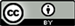1中国人民解放军战略支援部队航天系统部，北京

2北京神剑天军医学科学院华北祥鹄微波化学联合实验室，北京1. 前言

2. 社会生物学胡氏约等式

3. 宇宙化学元素个数的上限

4. H数138的奇妙性

138是宇宙中化学元素原子序数的极限，这个138还具有许多奇妙特性，人们称之为H数。我们且不说在138亿年前，在巨大的爆炸声中，现今的宇宙就诞生了，因此宇宙的年龄是138亿年。

46 × 3 = 138

34.5 × 4 = 138

13.8 × 10 = 138

6.9 × 20 = 138

5.52 × 25 = 138

4.6 × 30 = 138

3 × 46 = 138

2.3 × 60 = 138

1 × 138 = 138

0.05 × 2760 = 138

9.2 × 15 = 138

《千桥飞梦——胡文祥学习研究成果实录》第三章第七节社会生物学中，描述了莫里斯规律、邓巴定律、社交网站规律和学术交流规律都表明130至150是人类密友数量的极限。这一现代交往人数与原始部落的人数(猿人洞里的人数)相差无几，与早期社会村落里的人数也差不了多少，与多数动物单群里的个数也相差不大，这就是著名的胡氏约等式的由来，这是一条奇妙的社会生物学定律。

138 = 2 × 3 × 23

5. 结语